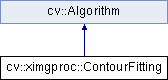OpenCV  3.4.0 Open Source Computer Vision
cv::ximgproc::ContourFitting Class Reference

Class for ContourFitting algorithms. ContourFitting match two contours $$z_a$$ and $$z_b$$ minimizing distance

$d(z_a,z_b)=\sum (a_n - s b_n e^{j(n \alpha +\phi )})^2$

where $$a_n$$ and $$b_n$$ are Fourier descriptors of $$z_a$$ and $$z_b$$ and s is a scaling factor and $$\phi$$ is angle rotation and $$\alpha$$ is starting point factor adjustement. More...

#include "fourier_descriptors.hpp"

Inheritance diagram for cv::ximgproc::ContourFitting:## Public Member Functions

ContourFitting (int ctr=1024, int fd=16)
Fit two closed curves using fourier descriptors. More details in  and . More...

void estimateTransformation (InputArray src, InputArray dst, OutputArray alphaPhiST, double *dist=0, bool fdContour=false)
Fit two closed curves using fourier descriptors. More details in  and . More...

void estimateTransformation (InputArray src, InputArray dst, OutputArray alphaPhiST, double &dist, bool fdContour=false)
Fit two closed curves using fourier descriptors. More details in  and . More...

int getCtrSize ()

int getFDSize ()

void setCtrSize (int n)
set number of Fourier descriptors used in estimateTransformation More...

void setFDSize (int n)
set number of Fourier descriptors when estimateTransformation used vector<Point> More...Public Member Functions inherited from cv::Algorithm
Algorithm ()

virtual ~Algorithm ()

virtual void clear ()
Clears the algorithm state. More...

virtual bool empty () const
Returns true if the Algorithm is empty (e.g. in the very beginning or after unsuccessful read. More...

virtual String getDefaultName () const

virtual void read (const FileNode &fn)
Reads algorithm parameters from a file storage. More...

virtual void save (const String &filename) const

virtual void write (FileStorage &fs) const
Stores algorithm parameters in a file storage. More...

void write (const Ptr< FileStorage > &fs, const String &name=String()) const
simplified API for language bindings This is an overloaded member function, provided for convenience. It differs from the above function only in what argument(s) it accepts. More...Static Public Member Functions inherited from cv::Algorithm
template<typename _Tp >
static Ptr< _Tp > load (const String &filename, const String &objname=String())
Loads algorithm from the file. More...

template<typename _Tp >
static Ptr< _Tp > loadFromString (const String &strModel, const String &objname=String())
Loads algorithm from a String. More...

template<typename _Tp >
static Ptr< _Tp > read (const FileNode &fn)
Reads algorithm from the file node. More...Protected Member Functions inherited from cv::Algorithm
void writeFormat (FileStorage &fs) const

## Detailed Description

Class for ContourFitting algorithms. ContourFitting match two contours $$z_a$$ and $$z_b$$ minimizing distance

$d(z_a,z_b)=\sum (a_n - s b_n e^{j(n \alpha +\phi )})^2$

where $$a_n$$ and $$b_n$$ are Fourier descriptors of $$z_a$$ and $$z_b$$ and s is a scaling factor and $$\phi$$ is angle rotation and $$\alpha$$ is starting point factor adjustement.

## § ContourFitting()

 cv::ximgproc::ContourFitting::ContourFitting ( int ctr = 1024, int fd = 16 )
inline

Fit two closed curves using fourier descriptors. More details in  and .

Parameters
 ctr number of Fourier descriptors equal to number of contour points after resampling. fd Contour defining second shape (Target).

## § estimateTransformation() [1/2]

 void cv::ximgproc::ContourFitting::estimateTransformation ( InputArray src, InputArray dst, OutputArray alphaPhiST, double * dist = 0, bool fdContour = false )
Python:
alphaPhiST, dist=cv.ximgproc_ContourFitting.estimateTransformation(src, dst[, alphaPhiST[, fdContour]])

Fit two closed curves using fourier descriptors. More details in  and .

Parameters
 src Contour defining first shape. dst Contour defining second shape (Target). alphaPhiST : $$\alpha$$=alphaPhiST(0,0), $$\phi$$=alphaPhiST(0,1) (in radian), s=alphaPhiST(0,2), Tx=alphaPhiST(0,3), Ty=alphaPhiST(0,4) rotation center dist distance between src and dst after matching. fdContour false then src and dst are contours and true src and dst are fourier descriptors.

## § estimateTransformation() [2/2]

 void cv::ximgproc::ContourFitting::estimateTransformation ( InputArray src, InputArray dst, OutputArray alphaPhiST, double & dist, bool fdContour = false )
Python:
alphaPhiST, dist=cv.ximgproc_ContourFitting.estimateTransformation(src, dst[, alphaPhiST[, fdContour]])

Fit two closed curves using fourier descriptors. More details in  and .

Parameters
 src Contour defining first shape. dst Contour defining second shape (Target). alphaPhiST : $$\alpha$$=alphaPhiST(0,0), $$\phi$$=alphaPhiST(0,1) (in radian), s=alphaPhiST(0,2), Tx=alphaPhiST(0,3), Ty=alphaPhiST(0,4) rotation center dist distance between src and dst after matching. fdContour false then src and dst are contours and true src and dst are fourier descriptors.

## § getCtrSize()

 int cv::ximgproc::ContourFitting::getCtrSize ( )
inline
Python:
retval=cv.ximgproc_ContourFitting.getCtrSize()
Returns
number of fourier descriptors

## § getFDSize()

 int cv::ximgproc::ContourFitting::getFDSize ( )
inline
Python:
retval=cv.ximgproc_ContourFitting.getFDSize()
Returns
number of fourier descriptors used for optimal curve matching

## § setCtrSize()

 void cv::ximgproc::ContourFitting::setCtrSize ( int n )
Python:
None=cv.ximgproc_ContourFitting.setCtrSize(n)

set number of Fourier descriptors used in estimateTransformation

Parameters
 n number of Fourier descriptors equal to number of contour points after resampling.

## § setFDSize()

 void cv::ximgproc::ContourFitting::setFDSize ( int n )
Python:
None=cv.ximgproc_ContourFitting.setFDSize(n)

set number of Fourier descriptors when estimateTransformation used vector<Point>

Parameters
 n number of fourier descriptors used for optimal curve matching.

The documentation for this class was generated from the following file:
• /build/master-contrib_docs-lin64/opencv_contrib/modules/ximgproc/include/opencv2/ximgproc/fourier_descriptors.hpp